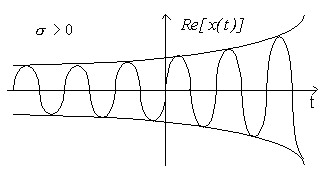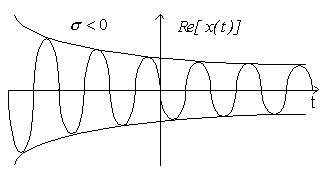# Signal Characteristics

### Even and Odd Functions

Any continuous time signal can be expressed as the sum of an even signal and an odd signal:

x(t) = xe(t) + xo(t)

 Even :    xe(t) = xe (-t) Odd:    xo(t) = -xo(-t)

An even signal is symmetric across the vertical axis.
An odd signal is anti-symmetric across the vertical axis.

 xe(t) =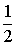(x(t) + x(-t)) xo(t) =(x(t) - x(-t))

Example, given the unit step function (a discontinuous continuous-time signal),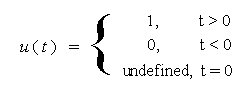find ue(t) and uo(t)

### Periodic Functions

How can we tell if a continuous- time signal x(t) is periodic? That is, given t and T, is there some period T >0 such that

x(t) = x(t + T)?

If x(t) is periodic with period T, it is also periodic with period nT, that is:

x(t) = x(t + nT).

The minimum value of T that satisfies x(t) = x(t + T) is called the fundamental period of the signal and we denote it as T0.

The fundamental frequency of the signal in hertz (cycles/second) is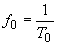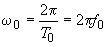If x1(t) is periodic with period T1 and x2(t) is periodic with period T2, then the sum of the two signals x1(t) + x2(t) is periodic with period equal to the least common multiple(T1, T2) if the ratio of the two periods is a rational number, i.e.: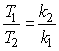Let   T '  =   k1T1  =  k2T2, and z(t) = x1(t) + x2(t),

z(t + T ' ) =  x1(t +  k1T1) +  x2(t +  k2T2) =  x1(t) + x2(t) =  z(t)

Examples of periodic signals are infinite sine and cosine waves.

Examples: Given x1(t) = cos(3t), and x2(t) = sin(5t).
find the period of x1(t)+ x2(t) or state that it is aperiodic.

Examples: Given x1(t) = cos(6t), and x2(t) = sin(8t).
find the period of x1(t)+ x2(t) or state that it is aperiodic.

Examples: Given x1(t) = cos(t), and x2(t) = sin(πt).
find the period of x1(t)+ x2(t) or state that it is aperiodic.

# Common Signals in Engineering

### Exponential Signals

x(t) = C eat,  C and a can be complex.

a  = σ + 0, σ =  real part, ω0 =  imaginary part.

These are very important because complex exponential are "eigenfunctions" of LTI systems:

As we will see, if you input x(t) = eat to a linear time-invariant system, we will get y(t) = H(a)eat as the output.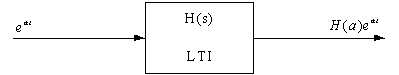*** EULER's FORMULA *** - Memorize:

e  =  cosθ  + jsinθ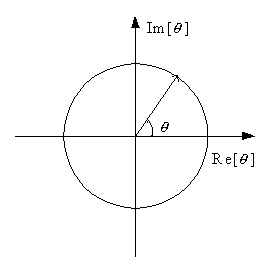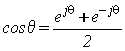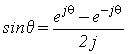1.)   Case 1, C and a real, x(t) = Ceat

a = σ > 0.
GROWING exponential,
Chemical reactions, uninhibited growth of bacteria (as might be found if the potato salad is left out too long), human population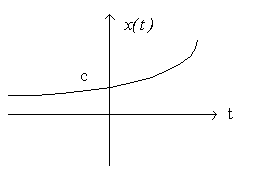a = σ < 0.
DECAYING exponential
Radioactive decay, RC circuit response, damped ME system.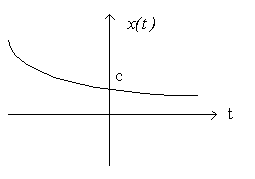a = σ = 0 => x(t) is constant (DC) signal.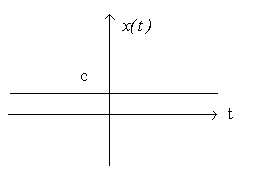2.)   Case 2, C complex, and a imaginary,
a is purely imaginary (σ = 0), a = 0

C = Ae where A and φ are real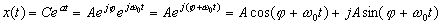x(t) is a complex sinusoid.

If C is real (φ=0), then x(t) = Acosω0t + jAsinω0t.
In both cases, x(t) is periodic, i.e.

x(t) = x(t + T) where T is the period.

Why is x(t) periodic?

 x(t) =x(t + T) =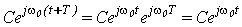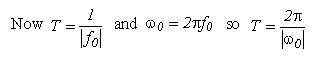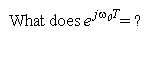A real sinusoid: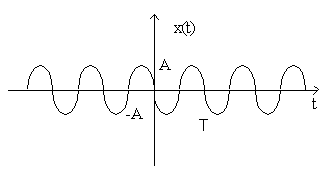T is the fundamental period

3.)   Case 3

In the most general case, C and a are complex:
 C =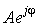x(t) =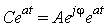=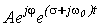=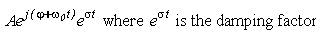=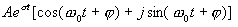The real part is  Aeσtcos(ω0t  +  φ)

The imaginary part is  Aeσtsin(ω0t  +  φ)

Example: Plot real part of this signal for σ > 0 and σ < 0